Processing ......FreeComputerBooks.com Links to Free Computer, Mathematics, Technical Books all over the World

O'Reilly® Think Bayes: Bayesian Statistics in Python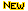Top Free Computer Networking Books - 100% Free or Open Source!
• Title: Think Bayes: Bayesian Statistics in Python
• Author(s) Allen B. Downey
• Publisher: O'Reilly Media; 2nd edition (June 15, 2021); eBook (CC Edition by Green Tea Press)
• Paperback: 338 pages
• eBook: HTML, PDF, ePub, Kindle, etc.
• Language: English
• ISBN-10: 149208946X
• ISBN-13: 978-1492089469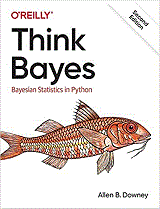Book Description

If you know how to program, you're ready to tackle Bayesian statistics. With this book, you'll learn how to solve statistical problems with Python code instead of mathematical formulas, using discrete probability distributions rather than continuous mathematics. Once you get the math out of the way, the Bayesian fundamentals will become clearer and you'll begin to apply these techniques to real-world problems.

Bayesian statistical methods are becoming more common and more important, but there aren't many resources available to help beginners. Based on undergraduate classes taught by author Allen B. Downey, this book's computational approach helps you get a solid start.

• Use your existing programming skills to learn and understand Bayesian statistics
• Work with problems involving estimation, prediction, decision analysis, evidence, and hypothesis testing
• Get started with simple examples, using coins, dice, and a bowl of cookies, M&Ms, Dungeons & Dragons dice, paintball, and hockey
• Learn computational methods for solving real-world problems, such as interpreting SAT scores, simulating kidney tumors, and modeling the human microbiome.
• Allen Downey is a Professor of Computer Science at Olin College of Engineering. He has taught computer science at Wellesley College, Colby College and U.C. Berkeley. He has a Ph.D. in Computer Science from U.C. Berkeley and Master’s and Bachelor’s degrees from MIT. He is the author of Think Python, Think Bayes, Think DSP, and a blog, Probably Overthinking It.
Reviews, Ratings, and Recommendations: Related Book Categories: Read and Download Links:Similar Books:
•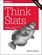Think Stats, 2nd Edition: Exploratory Data Analysis in Python

This concise introduction shows you how to perform statistical analysis computationally, rather than mathematically, with programs written in Python. You'll become familiar with distributions, the rules of probability, visualization, and many other tools and concepts.

•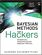Bayesian Methods for Hackers: Probabilistic Programming

This book illuminates Bayesian inference through probabilistic programming with the powerful PyMC language and the closely related Python tools NumPy, SciPy, Matplotlib, through practical examples and computation - no advanced mathematics required.

•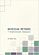Bayesian Methods for Statistical Analysis (Borek Puza)

Bayesian methods for statistical analysis is a book on statistical methods for analysing a wide variety of data. It contains many exercises, all with worked solutions, including complete computer code.

•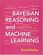Bayesian Reasoning and Machine Learning (David Barber)

This practical introduction is ideally suited to computer scientists without a background in calculus and linear algebra. You'll develop analytical and problem-solving skills that equip them for the real world. Numerous examples and exercises are provided.

•An Introduction to Bayesian Thinking (Merlise Clyde, et al.)

This book provides an introduction to Bayesian inference in decision making without requiring calculus. It may be used on its own as an open-access introduction to Bayesian inference using R for anyone interested in learning about Bayesian statistics.

•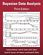Bayesian Data Analysis (Andrew Gelman, et al.)

This classic book is widely considered the leading text on Bayesian methods, lauded for its accessible, practical approach to analyzing data and solving research problems. It takes an applied approach to analysis using up-to-date Bayesian methods.

•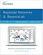Bayesian Networks and BayesiaLab (Stefan Conrady, et al.)

This practical introduction is geared towards scientists who wish to employ Bayesian Networks for applied research using the BayesiaLab software platform. It can serve as a self-study guide for learners and as a reference manual for advanced practitioners.

Book Categories
 :All CategoriesTop BooksRecent BooksMiscellaneous BooksComputer LanguagesComputer ScienceData Science/DatabasesElectrical EngineeringJava and Java EE (J2EE)Linux and UnixMathematicsMicrosoft and .NETMobile ComputingNetworking and CommunicationsSoftware EngineeringSpecial TopicsWeb Programming
Other Categories